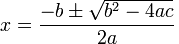Posted in Science & Nature

## Arithmetic

Although we all learn mathematics to a high level during our schooling years, most of us find that as working adults, we lose much of our maths skills due to lack of practice. This may be fine for advanced concepts such as calculus and matrices, but we tend to forget even the most basic arithmetic skills, instead choosing to rely on calculators on our phones and computers.

But maths is all around us in day-to-day life. From figuring out how much you save on a sale, to splitting a bill, to calculating tips when you travel in the USA, arithmetic is a handy life skill that many of us have forgotten. As easy as it is to pull out your phone and use the calculator app, here are a few tips to improve your arithmetic skills for quick mental calculations.

If you need to multiply a 2-digit number (e.g. 12 x 17), divide one of the number into its 10’s and 1’s, multiply the other number to each of these numbers then add them.

(e.g. (12 x 10) + (12 x 7) = 120 + 84 = 204)

You can further subdivide the numbers to break it down into easy bite-sized calculations.

e.g. 34 x 26 = (34 x 20) + (34 x 6) = (34 x 2 x 10) + ((30 x 6) + (4 x 6)) = 680 + (180 + 24) = 884

When adding or subtracting large numbers, use 10’s and 100’s for easier calculations. Essentially, you can “fill in the gap” up or down to the nearest 10’s or 100’s, then add/subtract the remainder.

e.g. 64 + 13 -> take 6 away from 13 and add to 64 -> 70 + 7 = 77

You can do this in multiple steps to break a complicated addition or subtraction into simple maths.

Learn to manipulate the decimal point to make multiplication and division simpler. 20% of 68.90 sounds difficult, but if you understand how the decimal point works, you can simply multiply 2 then divide by 10 to get the answer.

e.g. 68.90 x 2 =137.80 / 10 = 13.78

An extension of this is learning basic fractions, such as knowing that 0.5 is half and 0.2 is one-fifth.

e.g. 32 x 15 = 32 x (1.5 x 10) -> so you can add half of 32 to itself (x1.5) then x10 -> 48 x 10 = 480

Lastly, a handy mathematic trick is knowing that X% of Y = Y% of X. This means that if one side of the equation is easier, you can convert it easily. For example, 4% of 25 sounds much more difficult than 25% of 4 (or quarter of 4), yet the answer is the same.

The common theme of these tips is using shortcuts and breaking down complicated equations into bite-sized steps so that your brain can solve simple arithmetic in sequence. This may be asking for too much in a time when all of us seem to have minimal attention spans, but you never know when basic maths will come in handy.

Posted in Science & Nature